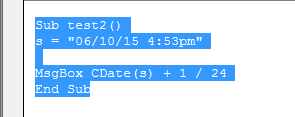### How to add time/hours together in Excel VBA?”

Let’s us start from the basic declarations in Excel. We can declare the date variable as follows:

`Dim date1 as Date`

Now we can pass a date to this variable as

`date1 = "11/03/2020"`

But we can also pass the time along with date which will be saved in the variable type :

`date1 = "11/03/2020 4:53pm"`

Now we want to add hours to this variable which has got the date and time together

Suppose we want to add 1 hour to date1, we cannot directly add it like:

` Date1 = date1 + 1`

which is not correct.

So we are using the following function and sub for the same:

```Private Sub TestIt()
Public Function AddHour(ByVal sTime As String) As String
Dim dt As Date

dt = CDate(sTime)

End Function```In this function we are declaring the variable dt as date and then the value “06/10/15 4:53pm” is passed to this variable from the sub.

This function used an inbuilt function Dateadd to add 1 hour and then the result is formatted as the input format.

We can also use another simple way:

```Sub test2()
s = "06/10/15 4:53pm"

MsgBox CDate(s) + 1 / 24
End Sub```It will also give the same result.## Template

```Further reading: